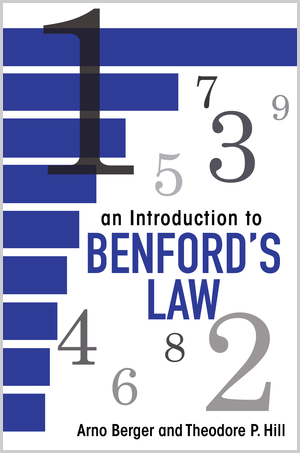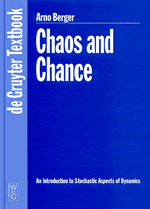# Books

• An Introduction to Benford's Law (with T.P. Hill), Princeton University Press, Princeton and Oxford, 2015.This book provides the first comprehensive treatment of Benford's law, the surprising logarithmic distribution of significant digits discovered in the late nineteenth century. Establishing the mathematical and statistical principles that underpin this intriguing phenomenon, the text combines up-to-date theoretical results with overviews of the law's colorful history, rapidly growing body of empirical evidence, and wide range of applications.

An Introduction to Benford's Law begins with basic facts about significant digits, Benford functions, sequences, and random variables, including tools from the theory of uniform distribution. After introducing the scale-, base-, and sum-invariance characterizations of the law, the book develops the significant-digit properties of both deterministic and stochastic processes, such as iterations of functions, powers of matrices, differential equations, and products, powers, and mixtures of random variables. Two concluding chapters survey the finitely additive theory and the flourishing applications of Benford's law.

Carefully selected diagrams, tables, and close to 150 examples illuminate the main concepts throughout. The text includes many open problems, in addition to dozens of new basic theorems and all the main references. A distinguishing feature is the emphasis on the surprising ubiquity and robustness of the significant-digit law. This text can serve as both a primary reference and a basis for seminars and courses.

Publisher's site (opens a new window or tab)

• Chaos and Chance, An Introduction to Stochastic Aspects of Dynamics, deGruyter Textbook, deGruyter, Berlin-New York, 2001.With emphasis on stochastic aspects of deterministic systems this short book introduces the reader to the basic facts and some special topics of applied ergodic theory. It adresses advanced undergraduate and graduate students from various disciplines, i.e. mathematicians, physicists, electrical and mechanical engineers. Based upon a sound (but non-technical) mathematical introduction, a number of typical examples from applications (mostly from mechanics) are thoroughly discussed. By studying both probabilistic and deterministic features of dynamical systems the reader will develop what might be considered a unified view on chaos and chance as two sides of the same thing.

Publisher's site (opens a new window or tab)

# Articles

• A beautiful inequality by Saint-Venant and Polya revisited, to appear in Amer. Math. Monthly (2023). (PDF, 178KB)
•
• On planar curves with position-dependent curvature, to appear in J. Dynam. Differential Equations (2022). (PDF, 2MB)
•
• A note on the distributions of (log n) mod 1, Unif. Distrib. Theory 17 (2022), 77-100. (PDF, 777KB)
•
• Intermittent synchronization in finite-state random networks under Markov perturbations (with H. Qian, S. Wang and Y. Yi), Comm. Math. Phys. 384 (2021), 1945-1970. (PDF, 689KB)
•
• The mathematics of Benford's law: a primer (with T.P. Hill), Stat. Methods Appl. 30 (2021), 779-795. (PDF, 689KB)
•
• Asymptotics of one-dimensional Levy approximations (with C. Xu), J. Theoret. Probab. 33 (2020), 1164-1195. (PDF, 758KB)
•
• On the classification of finite-dimensional linear flows (with A. Wynne), J. Dynam. Differential Equations 32 (2020), 23- 59. (PDF, 952KB)
•
• Best finite approximations of Benford's Law (with C. Xu), J. Theoret. Probab. 32 (2018), 1525-1553. (PDF, 1MB)
•
• Best finite constrained approximations of one-dimensional probabilities (with C. Xu), J. Approx. Theory 224 (2019), 1-36. (PDF, 598KB)
•
• On the significands of uniform random variables (with I. Twelves), J. Appl. Probab. 55 (2018), 353-367. (PDF, 231KB)
•
• On linear dependence of trigonometric numbers, Carpatian J. Math. 34 (2018), 157-166. (PDF, 284KB)
•
• Global saddle-type dynamics for convex second-order difference equations (with A. Duh), J. Difference Equ. Appl. 23 (2017), 1807-1823. (PDF, 496KB)
•
• More grade school triangles, Amer. Math. Monthly 124 (2017), 324-336. (PDF, 724KB)
•
• What is . . . Benford's law? (with T.P. Hill) Notices Amer. Math. Soc. 64 (2017), 132-134. (PDF, 129KB)
•
• A characterization of Benford's Law in discrete-time linear systems (with G. Eshun), J. Dynam. Differential Equations 28 (2016), 431-469. (PDF, 447KB)
•
• Most linear flows on Rd are Benford, J. Differential Equations 259 (2015), 1933-1957. (PDF, 289KB)
•
• Benford solutions of linear difference equations (with G. Eshun), in: Z. AlSharawi et al. (eds.), Theory and Applications of Difference Equations and Discrete Dynamical Systems, Springer Proceedings in Mathematics & Statistics 102 (2014), 23-60. (PDF, 884KB)
•
• Invariant measures for general induced maps and towers (with R. Zweimüller), Discrete Contin. Dyn. Syst. 33 (2013), 3885-3901. (PDF, 478KB)
•
• A limit theorem for occupation measures of Lévy processes in compact groups (with S.N. Evans), Stoch. Dyn. 13 (2013), 1250008, 16 pp. (PDF, 291KB)
•
• A basic theory of Benford's law (with T.P. Hill), Probab. Surv. 8 (2011), 1-126. (online)
•
• Finite-state Markov chains obey Benford's law (with T.P. Hill, B. Kaynar and A. Ridder), SIAM J. Matrix Anal. Appl. 32 (2011), 665-684. (PDF, 291KB)
•
• Some dynamical properties of Benford sequences, J. Difference Equ. Appl. 17 (2011), 137-159. (PDF, 323KB)
•
• Benford's law strikes back: no simple explanation in sight for mathematical gem (with T.P. Hill), Math. Intelligencer 33 (2011), 85-91. (PDF, 333KB)
•
• On finite-time hyperbolicity, Commun. Pure Appl. Anal. 10 (2011), 963-981. (PDF, 323KB)
•
• More on finite-time hyperbolicity, Bol. Soc. Esp. Mat. Apl. SeMA 51 (2010), 25-32. (PDF, 182KB)
•
• An improved maximum allowable transfer interval for Lp-stability of networked control systems (with A. Jentzen, F. Leber, D. Schneisgen and S. Siegmund), IEEE Trans. Automat. Control 55 (2010), 179-184. (PDF, 170KB)
•
• A definition of spectrum for differential equations on finite time (with T.S. Doan and S. Siegmund), J. Differential Equations 246 (2009), 1098-1118. (PDF, 711KB)
•
• Scale-distortion inequalities for mantissas of finite data sets (with T.P. Hill and K.E. Morrison), J. Theoret. Probab. 21 (2009), 97-117. (PDF, 414KB)
•
• Nonautonomous finite-time dynamics (with T.S. Doan and S. Siegmund), Discrete Contin. Dyn. Syst. Ser. B. 9 (2008), 463-492. (PDF, 787KB)
•
• Counting uniformly attracting solutions of nonautonomous differential equations, Discrete Contin. Dyn. Syst. Ser. S 1 (2008), 15-25. (PDF, 187KB)
•
• Uniformly attracting solutions of nonautonomous differential equations (with S. Siegmund), Nonlinear Analysis 68 (2008), 3789-3811. (PDF, 833KB)
•
• On the distribution of mantissae in nonautonomous difference equations (with S. Siegmund), J. Difference Equ. Appl. 13 (2007), 829-845. (PDF, 230KB)
•
• Newton's Method obeys Benford's Law (with T.P. Hill), Amer. Math. Monthly 114 (2007), 588-601. (PDF, 4.76MB)
•
• A Characterisation of Newton maps (with T.P. Hill), ANZIAM J. 48 (2006), 1-13. (PDF, 112KB)
•
• Chaos in spatially extended systems via the Peak-Crossing Bifurcation (with L. Bunimovich), Internat. J. Bifur. Chaos Appl. Sci. Engrg. 15 (2005), 11, 1-15. (PDF, 983KB)
•
• Benford's Law in power-like nonautonomous dynamical systems, Stoch. Dyn 5 (2005), 1-21. (PDF, 305KB)
•
• One-dimensional dynamical systems and Benford's law (with L. Bunimovich and T.P. Hill), Trans. Amer. Math. Soc. 357 (2005), 197-219. (PDF, 340KB)
•
• Multi-dimensional dynamical systems and Benford's law, Discrete Contin. Dyn. Syst. Ser. A 13 (2005), 219-237. (PDF, 311KB)
•
• Almost automorphic dynamics in symbolic lattices (with S. Siegmund and Y. Yi), Ergodic Theory Dyn. Syst. 24 (2004), 677-696. (PDF, 255KB)
•
• A criterion for non-persistence of travelling breathers for perturbations of the Ablowitz-Ladik lattice (with R.S. MacKay and V.M. Rothos), Discrete Contin. Dyn. Syst. Ser. B 4 (2004), 911-920. (PDF, 201KB)
•
• On the gap between random dynamical systems and continuous skew products, (with S. Siegmund), J. Dynam. Differential Equations 15 (2003), 237-279. (PDF, 515KB)
•
• Propagation of small waves in inextensible strings (with M. Schagerl), Wave Motion 35 (2001), 339-353. (PDF, 453KB)
•
• Rigorous error bounds for RK methods in the proof of chaotic behaviour, J. Comput. Appl. Math. 111 (1999), 13-24. (PDF, 208KB)
•

# Other publications and work in progress

• Linear independence of trigonometric numbers, preprint (arXiv).
•
• A Short Introduction to the Mathematical Theory of Benford's Law (with T.P. Hill), pp. 22-66 in: S.J. Miller (ed.), The Theory and Applications of Benfords Law, Princeton University Press, Princeton and Oxford, 2015. (PDF, 773KB, book website)
•
• Fundamental Flaws in Feller's Classical Derivation of Benford's Law (with T.P. Hill), preprint. (PDF, 99KB)
•
• Large spread does not imply Benford's Law, preprint. (PDF, 96KB)
•
• Dynamics and Digits: On the Ubiquity of Benford's Law, Proceedings of Equadiff 2003, World Scientific, Singapore, 2005, 693-695. (PDF, 82KB)
•
• On the appropriate treatment of singularly perturbed wave equations (with M. Schagerl), Z. Angew. Math. Mech. 81 (2001), 623-624.
•
• On the regular and chaotic motion of a kicked pendulum: a Markovian approach, Z. Angew. Math. Mech. 81 (2001), 611-612. (PDF, 280KB)
•
• Zur Stabilität eines Doppelpendels mit geradlinig geführtem Endpunkt, Z. Angew. Math. Mech. 80 (2000), 335-336. (PDF, 2.34MB)
•
• Zum praktischen Nachweis von Chaos mit Hilfe der Conley Index Theorie, Z. Angew. Math. Mech. 79 (1999), 791-792. (PDF, 297KB)
•
• RK methods and the proof of chaotic behaviour, Proc. of the Second Meeting on Numerical Methods for Differential Equations, Coimbra, 1998. (PDF, 7.57MB)
•

# Book reviews

• R. Taschner: The Continuum, Book review, to appear in Z. Angew. Math. Mech., 2007. (PDF, 41KB)
•
• M. Denker: Einführung in die Analysis dynamischer Systeme, Book review, Z. Angew. Math. Mech. 86 (2006), 251-252. (PDF, 45KB)
•
• T. Kaczynski, M. Mischaikow, M. Mrozek: Computational Homology, Book review, Z. Angew. Math. Mech. 86 (2006), 334-335. (PDF, 45KB)
•
• G. H. Choe: Computational Ergodic Theory, Book review, Z. Angew. Math. Mech. 86 (2006), 743-744. (PDF, 53KB)